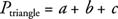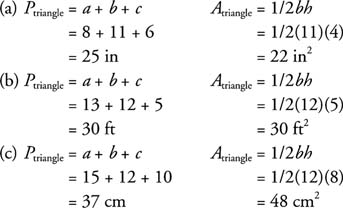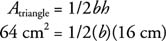## Triangles

Look at Δ ABD in Figure 1. If a line BC is drawn through B parallel to AD and another line DC is drawn through D parallel to AB , then you will have formed a parallelogram. BD is now a diagonal in this parallelogram. Because a diagonal divides a parallelogram into two congruent triangles, the area of Δ ABD is exactly half the area ofABCD.Figure 1 Area of a triangle is half the area of the associated parallelogram.

## Finding the Area

Because A parallelogram = bh, then## Finding the Perimeter

In Δ ABD (Figure 1), the perimeter is found simply by adding the lengths of the three sides.Example 1: Find the perimeter and area for the triangles in Figures (a), (b), and (c).Figure 2 Finding perimeters and areas of triangles.Example 2: If the area of a triangle is 64 cm2 and it has a height of 16 cm, find the length of its base.Multiply both sides by 2.The triangle will have a base of 8 centimeters.